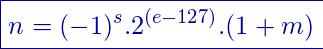# Floating Point Representation

## Introduction

In this arti­cle we are going to see what is a float­ing point and how dec­i­mal val­ues are rep­re­sent­ed using this system.

Float­ing point rep­re­sen­ta­tion is a way of writ­ing a dec­i­mal num­ber that resem­bles sci­en­tif­ic nota­tion. This allows us to rep­re­sent and oper­ate with very large num­bers and also with very small num­bers (with many decimals).

The float­ing point com­put­ing stan­dard is described in IEEE 754.

# MOST SEARCHED VIDEOS FROM MY CHANNEL

## Structure of a floating point number

This rep­re­sen­ta­tion sys­tem uses a cer­tain num­ber of bina­ry dig­its depend­ing on the type of accu­ra­cy (com­mon­ly 16, 32, 64 and 128 bits).

A bit is des­tined to the sign, i.e. if that bit is worth 0 it is a pos­i­tive num­ber, if it is worth 1 it is a neg­a­tive number.

The remain­ing bits are dis­trib­uted in the rep­re­sen­ta­tion of the dec­i­mals (usu­al­ly called man­tis­sa) and the exponent.In the expres­sion n it is the dec­i­mal num­ber to represent.

The let­ter s is the bit for the sign (if s is 0, the expres­sion (-1) raised to 0 results in 1 positive).

The let­ter e is the expo­nent and m is the mantissa.

## Move decimal numbers to floating point

1- Take the num­ber to rep­re­sent, sep­a­rate the sign and write the absolute val­ue in base 2.

2- The absolute val­ue in base 2 is writ­ten in sci­en­tif­ic nota­tion in nor­mal­ized base 2.

3- The expo­nent is expressed in excess nota­tion (it will depend on the type of pre­ci­sion chosen).

4- The coef­fi­cient is writ­ten on the man­tis­sa with­out the whole part, because the nor­mal­iza­tion in step 2 forces the whole part of the man­tis­sa to be 1, stor­ing it does not pro­vide information.

## System Truncation Error Floating Point

A trun­ca­tion error occurs when you take a cer­tain num­ber of dig­its from one num­ber and leave out the others.

Think of the num­ber π (3.14159265…), which is an irra­tional num­ber with infi­nite digits.

Com­put­ers can­not store infi­nite infor­ma­tion in mem­o­ry because infi­nite­ly large mem­o­ry would be need­ed, so at some point it must stop.

If we trun­cate all the dec­i­mal part to π and we are left with only 3, we will be mak­ing an error of approx­i­mate­ly 4.5% rel­a­tive to the real val­ue of π . If on the oth­er hand we take into account the first two dec­i­mals of π, we are left with 3.14. In this case we will be mak­ing an error of approx­i­mate­ly 0.05% rel­a­tive to the real val­ue of π.

This error will occur in the float­ing point sys­tem either because we want to rep­re­sent irra­tional num­bers or because the dec­i­mal we want to rep­re­sent becomes irra­tional when passed to the bina­ry sys­tem (exam­ple 0.1 dec­i­mal has infi­nite dig­its when passed to binary).

Scroll to Top
Secured By miniOrange